|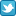Twitter |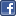Facebook |Google+ |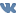VKontakte | LinkedIn|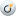Viadeo | English | Français | Español | العربية |International Journal of Innovation and Applied Studies
ISSN: 2028-9324     CODEN: IJIABO     OCLC Number: 828807274     ZDB-ID: 2703985-7
 Tuesday 16 August 2022

# Connect with IJIAS

••••••••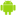Call for Papers - October 2022     |     Now IJIAS is indexed in EBSCO, ResearchGate, ProQuest, Chemical Abstracts Service, Index Copernicus, IET Inspec Direct, Ulrichs Web, Google Scholar, CAS Abstracts, J-Gate, UDL Library, CiteSeerX, WorldCat, Scirus, Research Bible and getCited, etc.

# Estimation of Errors: Mathematical Expressions of Temperature, Substrate Concentration and Enzyme Concentration based Formulas for obtaining intermediate values of the Rate of Enzymatic Reaction

Volume 2, Issue 2, February 2013, Pages 153–172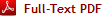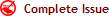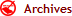Nizam Uddin1

Original language: English

Copyright © 2013 ISSR Journals. This is an open access article distributed under the Creative Commons Attribution License, which permits unrestricted use, distribution, and reproduction in any medium, provided the original work is properly cited.

# Abstract

This research paper is based on the estimation of errors in the formulas which are used to obtaining intermediate values of the rate of enzymatic reaction. The rate of enzymatic reaction is affected by concentration of substrate, Temperature, concentration of enzyme and other factors. The rise in Temperature accelerates an Enzyme reaction. At certain Temperature known as the optimum Temperature the activity is maximum. The concentration of substrate is the limiting factor, as the substrate concentration increases, the Enzyme reaction rate increases. Assuming a sufficient concentration of substrate is available, increasing Enzyme concentration will increase the enzymatic reaction rate. These formulas are derived from temperature, substrate concentration and enzyme concentration based mathematical functions. These formulas are used to obtaining intermediate values of the rate of enzymatic reaction. Formulas which are derived using Newton's method for interpolation are worked in conditions which are depending on the point lies. If the point lies in the upper half then used Newton's forward interpolation formula. If the point lies in the lower half then we used Newton's backward interpolation formula. And when the interval is not equally spaced then used Newton's divide difference interpolation formula. When the tabulated values of the function are not equidistant then used Lagrangian polynomial. Mathematical expressions are derived for estimation of errors using intermediate values and formulas. All expressions are worked in n limit which is the optimum limit.

Author Keywords: Estimation of Errors, Intermediate values, Mathematical Expressions, Rate of enzymatic reaction.